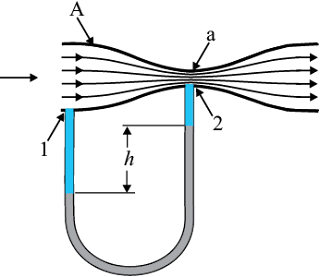# Venturi-meter

The Venturi-meter is a device to measure the flow speed of incompressible fluid. It consists of a tube with a broad diameter and a small constriction at the middle as shown in Fig. (1). A manometer in the form of a U-tube is also attached to it, with one arm at the broad neck point of the tube and the other at constriction as shown in Fig. (1).Fig. 1 A schematic diagram of Venturi-meter.

The manometer contains a liquid of density ρ$_m$ . The speed v$_1$ of the liquid flowing through the tube at the broad neck area A is to be measured from equation of continuity Eq. (10.10) the speed at the constriction becomes $v_2=\frac{A}{a}v_1$.

Then using Bernoulli’s equation (Eq.10.12) for (h$_1$ = h$_2$), we get

$P_1+\frac{1}{2}\rho v_1^2=P_2+\frac{1}{2}\rho v_1^2 \left(\frac{A}{a}\right)^2$

So that

$P_1-P_2=\frac{1}{2}\rho v_1^2 \left[\left(\frac{A}{a}\right)^2-1\right]$       (1)

This pressure difference causes the fluid in the U-tube connected at the narrow neck to rise in comparison to the other arm. The difference in height h measure the pressure difference.

$P_1-P_2=\rho_m gh=\frac{1}{2}\rho v_1^2 \left[\left(\frac{A}{a}\right)^2-1\right]$

So that the speed of fluid at wide neck is

$v_1=\sqrt{\frac{2\rho_m gh}{\rho \left[\left(\frac{A}{a}\right)^2-1\right]}}$   (2)

The principle behind this meter has many applications. The carburetor of automobile has a Venturi channel (nozzle) through which air flows with a high speed. The pressure is then lowered at the narrow neck and the petrol (gasoline) is sucked up in the chamber to provide the correct mixture of air to fuel necessary for combustion. Filter pumps or aspirators, Bunsen burner, atomisers and sprayers [See Fig. 2] used for perfumes or to spray insecticides work on the same principle.

Fig. 2 The spray gun. Piston forces air at high speeds causing a lowering of pressure at the neck of the container.

Example 1

Blood velocity: The flow of blood in a large artery of an anesthetised dog is diverted through a Venturi meter. The wider part of the meter has a cross-sectional area equal to that of the artery. A = 8 mm$^2$. The narrower part has an area a = 4 mm$^2$. The pressure drop in the artery is 24 Pa. What is the speed of the blood in the artery?

We take the density of blood from Table 10.1 to be 1.06 × 10$^3$  kg.m$^{-3}$.

The ratio of the areas is $\frac{A}{a}$ = 2.

Using Eq. (2) we obtain

$v_1=\sqrt{\frac{2 \times 24 \ Pa}{1060 \ kg.m^{-3} \times (2^2-1)}}$

v$_1$ = 0.123 m/s

Blood Flow and Heart Attack

Bernoulli’s principle helps in explaining blood flow in artery. The artery may get constricted due to the accumulation of plaque on its inner walls. In order to drive the blood through this constriction a greater demand is placed on the activity of the heart. The speed of the flow of the blood in this region is raised which lowers the pressure inside and the artery may collapse due to the external pressure. The heart exerts further pressure to open this artery and forces the blood through. As the blood rushes through the opening, the internal pressure once again drops due to same reasons leading to a repeat collapse. This may result in heart attack.